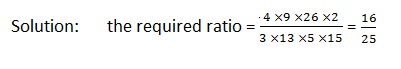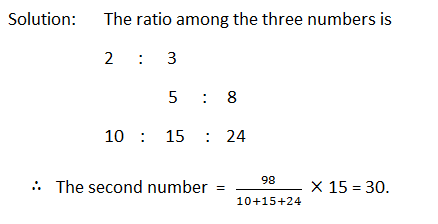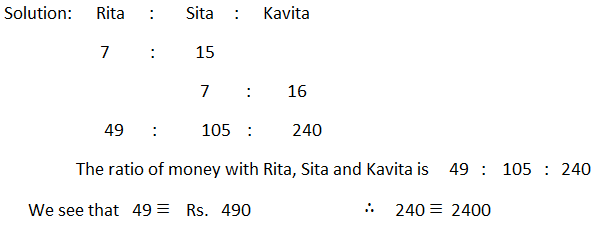New Student User - Use Code HELLO

# Ratio and Proportion - Rules with Examples

The number of times one quantity contains another quantity of the same kind is called the ratio of two quantities.

Clearly, the ratio of two quantities is equivalent to the fraction that one quantity is of the other.

Observe carefully that two quantities must be of the same kind.There can be a ratio between Rs. 20 and Rs. 30, but there can be no ratio between Rs.20 and 30 mangoes.

The ratio 2 to 3 is written as 2 : 3 or 2/3. 2 and 3 are called the terms of the ratio. 2 is the first term and 3 is the second term.

The first term of a ratio is called the antecedent and the second the consequent.

==>> Must read - Ratio and Proportions Tricks

COMPOUND RATIO
Ratios are compounded by multiplying together the antecedent for a new antecedent, and the consequents for a new consequent.

#### Ques 1.

Find the ratio compounded of the four ratios:
4 : 3, 9 : 13, 26 : 5 and 2 :15### INVERSE RATIO

1. If 2 : 3 be the given ratio, then 1/2  : 1/3 or 3 : 2 is called its inverse or reciprocal ratio.
2. If the antecedent =  the consequent, the ratio is called the ratio of equality, such as 3 : 3.
3. If the antecedent > the antecedent, the ratio is called the ratio of greater inequality, as 4 : 3.
4. If the antecedent < the consequent, the ratio is called the ratio of less inequality, as 3 : 4.

#### Ques 2.

Divide 1458 into two parts such that one may be to the other as 2 : 7.#### Ques 3.

Find three numbers in the ratio of 1 : 2 : 3, so that the sum of their squares is equal to 504.
Solution:       Let the numbers be x, 2x, 3x. Then we have,
⇒ X2 + (2x)2 + (3x)2 = 504  ⇒ 14x2 = 504
X = 6  Hence, the required numbers are 6, 12 and 18.

#### Ques 4.

The sum of three numbers is 98. If the ratio between the first number and second number be 2 : 3 and that between the second and third be 5 : 8, then find the second number.#### Ques 5.

The ratio of the money with Rita and sita is 7 : 15 and that with Sita and Kavita is 7 : 16. If Rita has Rs. 490, how much money does Kavita have ?## PROPORTION

Consider the two ratios:

1st ratio 2nd ratio

6  :  18 8  :  24

Since 6 is one-third of 18, and 8 is one-third 24, the two ratios are equal. The equality of ratio is called proportion.

The number 6, 18, 8 and 24 are said to be in proportion.

The proportion may be written as   6 : 18  :  :  8  : 24 (6 is to 18 as 8 is to 24)

Or,   6  : 18  = 8 : 24

Or, 6/18 =   8/24

If four quantities be in proportion, the product of the extremes is equal to the product of the means.
Let the four quantities 3, 4, 9 and 12 be in proportion,
We have     3/4 =   9/12Three quantities of the same kind are said to be in continued proportion when the ratio of the first to the second is equal to the ratio of the second to the third.

The second quantity is called mean proportion between the first and third; and the third quantity is called the third proportional to the first and second.

#### Ques 6.

Find the fourth proportional to the numbers 6, 8 and 15.
Solution:     If x be the fourth proportional, then  6 :  8  =  15   :   x
X = (8  ×  15 )/6 = 20